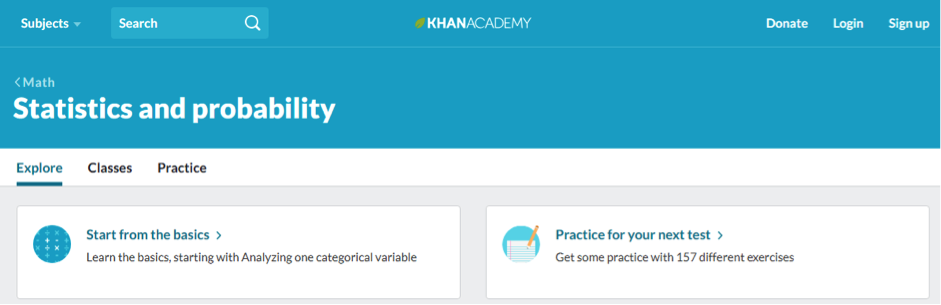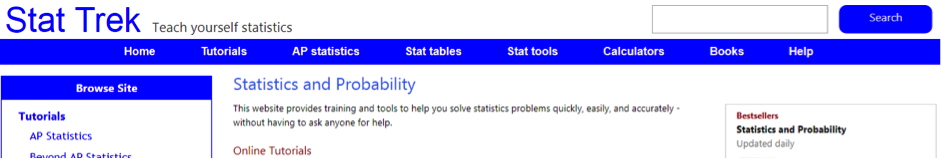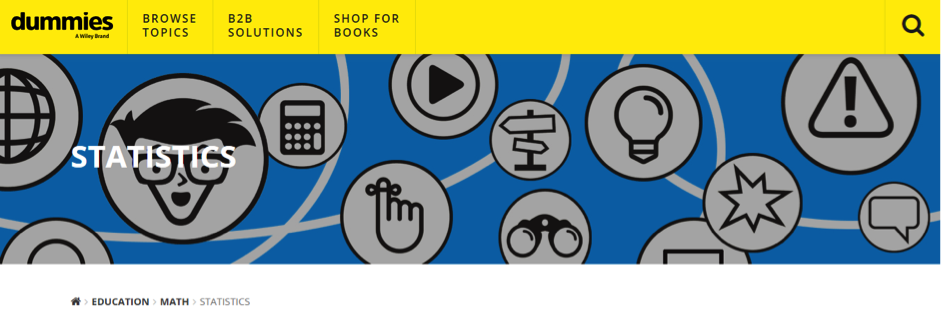# A+ For Statistics Homework: Nail It With 7 Magnificent Websites and Geeks’ HelpIn this article, you will find a comprehensive list of free online sources that will help you complete your statistics homework and get A+ as well as useful practical tips on learning statistics and doing statistics homework!

## Why is Statistics Important But so Challenging to Learn?

Statistics is used pretty much everywhere to analyze and make predictions, evaluate risks and develop strategies. Here is a list of some areas where statistics is applied:

• Weather forecasting
• Insurance risk evaluation
• Quality testing in production
• Disease effects and prediction in medicine
• Politics – election winner predictions
• Investment evaluation in business and finance
• Computer models
• Internet suggestions and search results

As you can see, statistics can be applied to pretty much any issue in science, industry, and sociology to collect, analyze, interpret, present, and organize data.

Most probably, you’re experiencing difficulties learning statistics because it is often taught out of context. In other words, learning formulas, terms, and theories is ineffective if you have no clue how they can be applied in practice. On top of all, statistical formulas often look like mathematical dinosaurs with multiple elements and can be intimidating. So, the first rule of completing a statistical homework is to remember that it is doable.

The second rule is to use great sources, so let’s get right into it!

## List of Online Statistics Homework Help SourcesThis source is one of the best and most used ones when it comes to online learning. The information is presented in form of short educational videos, where every concept and theory is explained using examples. This is extremely important because such method of explanation provides context for theory and allows you to understand what it all is about. You can also take free quizzes and tests to check your knowledge. Khan Academy will also help you learn to create and read graphs, which is extremely important in statistics.

• Easy to use and search
• Video explanations
• Examples
• Illustrations
• Free quizzes
• Free tests
• Creating charts and reading charts tutorials
• Learning tables and diagrams
• An extensive amount of information

### 2Stat TrekThis website provides info in several categories, including tutorials, AP statistics, statistics tables, calculators, and books. You will find not only explanations of any statistical term and theory, but examples and useful tables, which makes this source all the help you need to comple a statistics homework. Most importantly, you can use calculators to make calculations, graphs, and check your own calculations.

• Easy to navigate
• Contains extensive theory and tools
• Statistical tables available
• Explanations to every calculation
• Examples
• Contains matrix algebra and survey sampling tutorials
• Easy to understand, simple language
• Useful for learners with any level

Overall, this website is a statistics homework helper for completing statistics assignments. However, it does not help with good topics for your stats homework. Albert Gordy has collected unusual and non-cliché statistic project ideas for 2018-2019 — must check for everyone.

### 3Social Science StatisticsSocial Science Statistics has three major sections: statistical calculators, p-value calculators, and descriptive statistics. You can complete significance testing, tests of correlation, prediction, and calculate confidence intervals using statistical calculators in this source. P-value calculators will allow you to derive p-values from Z, t, chi-square, and Pearson (r). Finally, descriptive statistics tools allow calculating averages, variance, and make easy histogram and bar charts.

• Easy to navigate
• Great variety of calculators and tools
• Explanations of how calculators work
• Free quizzes with explanations of your results
• Tutorials
• Formulas
• Hypothesis testing

### 4Listen DataIf you need explanations of terms and concepts, this website is a good choice. Many articles contain full explanations as well as examples, which will help you understand the context and memorize better to apply while doing your statistics homework. If you are looking to learn basic, intermediate, or advanced statistics and need to find out about modeling and prediction, check this source out.

• Suitable for learners of all levels
• Easy to use
• Data visualization – highlighted sections of text
• Examples
• Tutorials
• Detailed explanations of theories and formulas

### 5Free Online Statistics Materials

This source is basically a free online statistics book. You’ll find extensive explanations, examples and definitions. That being said, there is no useless content here created just to take space – everything is to the point. The text is often organized in tables and lists, which makes it easier to read and search for whatever you need.

• Suitable for learners of all levels
• Easy to navigate
• Trustworthy
• Detailed explanations
• Examples
• Tutorials
• Forums for discussions

### 6Wiley’s Statistics for DummiesDespite that the title of this book and article series is weird, the content is definitely worth checking out. Wiley is a trustworthy source and provides online articles on a variety of statistics problems and terms with examples and detailed explanations. You can also check your own knowledge by solving small tests in each article, while correct answers are provided as well.

• Easy to understand explanations
• Variety of topics
• Examples
• Easy to find relevant content using a search tool

### 7Statistics Help for Students

The content of the website is definitely worth checking out. It contains useful guidelines explained in an easy way for students to get a clear understanding of some statistics terms. Make sure to visit this website if you need difficult terms to be explained in a good way.

## Tips and Tricks for Completing Statistics Homework

• Remember that in order to better understand statistics you need to learn it in context – try to search real examples of how certain theories are applied.
• If you come across any formula, make sure to write down every element of the formula to avoid mistakes and confusion while using it in the future
• If you’re experiencing issues applying formulas and statistical methods in practice, write down a step-by-step guideline of formula or method application, which will help you memorize the sequence of actions
• Use online calculators to make or check calculations and make sure you didn’t make any errors
• Take online quizzes and tests in statistics to check your knowledge and train
• Use examples of calculations to learn and complete your own statistics homework.
Stuck with homework?

get connected to a geekGetting homework help from a statistics Geek online is a stress-free solution as well. Type the title of the task you’re struggling with, then your course name and the deadline. Our system will find a Geek who can help you to nail any statistics homework and provide all the explanations you need.

This is a stress-free way of getting an A+ for your statistics homework!

### Popular articlesCongratulations! You have been successfully subscribed to Homework Lab blog!